## Wguide17M    Poynting Vector and Reverse Power Flow

Aug 10, 2017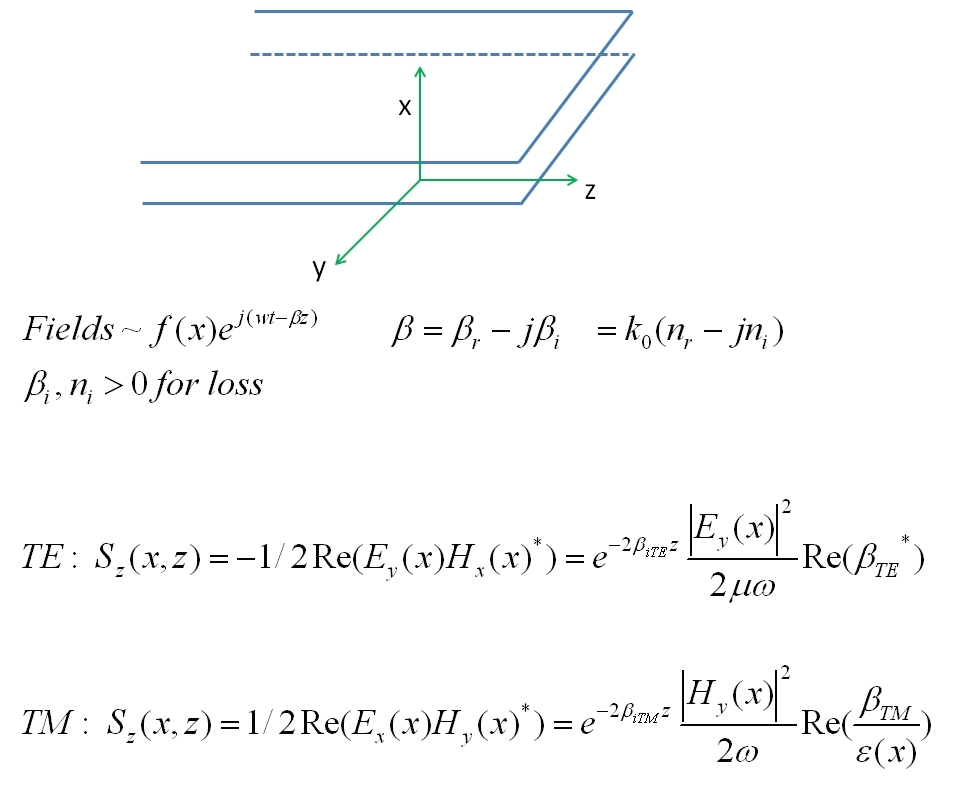where β is the complex propagation constant and the transverse field dependence f(x) = Ey(x) or Hy(x) for TE and TM modes respectively. These expressions for the time-averaged z component of Poynting vector (in the direction of phase propagation parallel to the layers) are general and apply to any layer with arbitrary complex dielectric permitivity ε(x) in a multi-layer slab waveguide where Ey(x) and Hy(x) depend on the mode solution β .

## Lossless Symmetric Waveguide

The time-averaged Poynting vector distributions for the TE0 and TM0 modes are shown below for a typical lossless simple symmetric slab waveguide. These distributions are independent of z since the mode is lossless. Note the discontinuity in Sz(x) for the TM case at the layer interfaces. This is a consequence of the discontinuity in the normal electric field component at the interfaces Ex(y) corresponding to the discontinuity in dielectric permittivity (or equivalently to the continuity of the displacement vector D at the interfaces of uniform bulk media in Maxwell's equations):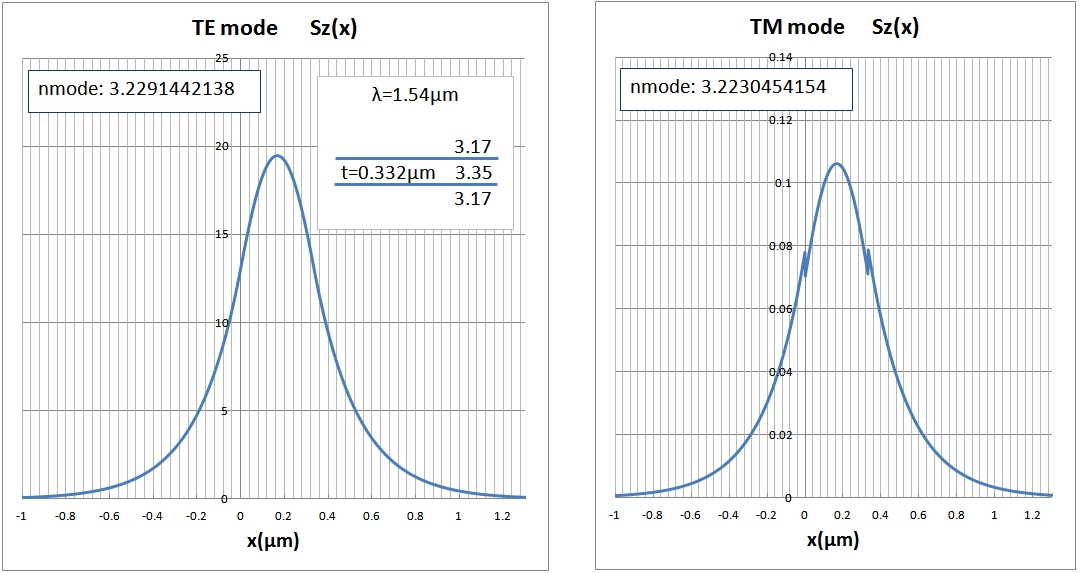The 2D plots below show all the field components and instantaneous (not time averaged) Poynting vector components at a fixed time. The instantaneous (and time-averaged) Poynting vector component Sz is discontinuous across the interfaces as mentioned above. The time-averaged Poynting vector is the average at any given (x,z) location of the instantaneous value and corresponds to the single line above (independent of z since there is no mode attenuation). The "islands" of instantaneous Poynting vector correspond to the peaks of EM energy density in the mode propagating along the +z direction without change with Hy and Ex being in phase. The component of power flow normal to the layers, Sx, is interesting. The TIME AVERAGED value of Sx is zero at any location, but the instantaneous flux shows a forward-reverse surging effect at the interfaces in the x direction with time as clearly seen in the distribution. This is obvious when one considers that the distributions shown simply move along the z propagation direction and for a fixed z, the Sx value changes in direction with the time-averaged value being identically zero, a consequence of the waveguide being lossless (compare the lossy surface-wave mode example below where the time averaged Sx flow is NOT zero). This is the same behaviour as the cyclic energy surge in the evanescent field present for the simple plane wave reflection for a Total Internal Reflection (TIR) case (Stratton 1941 p.499). For the present symmetric waveguide, this is not surprising as the field in the outer waveguide regions are essentially evanescent TIR-like fields. Note that the Sx peaks are displaced from the Sz peaks :## Lossy TM0 Surface Wave

The next example is the lossy interface "TM "surface wave" between a dielectric (~ GaAs) and a metal (Gold). Note that in the metal x<0, the time-averaged Poynting vector component in the z propagation direction is discontinuous at the interface, as in the simple example above, but in addition the time-averaged power flow within the metal (and the x-integrated power in the metal also) is in the REVERSE direction to the dominant power flow within the lossless GaAs dielectric which is in the +z phase velocity direction. The total net integrated (across the transverse direction x) power flow for both regions however is in the + z direction. The reverse power flow in the metal is a consequence of a sufficiently large negative real part of the dielectric constant of the metal (n^2), a typical feature of many metals at optical frequencies. The result below agrees exactly with Sharma et. al. El. Lett. V27 #5 Feb 1991 p.409:# Reverse Power Flow: Negative Permittivity Media

The general expressions above for the time-averaged Poynting vector Sz for TE and TM modes in a slab waveguide indicate under what conditions the time-averaged power flow in the mode propagation direction z may change sign (note that the instantaneous power flow may fluctuation in direction, as shown above for transverse power flow in the lossless waveguide mode case). For the TE case, with mode propagation in the + z direction, the direction of Sz in any layer is determined by Re(β*) which is simply β_re which is always positive for the assumed mode propagation direction. Therefore the time-averaged z component of the power flow in all layers for any TE slab mode, including lossy modes, will be in the +z phase velocity propagation direction.

The TM mode, as demonstrated above is considerably more "complex" in character depending on the layer dielectric constants. The sign of the Sz expression above for the general TM slab mode depends on the sign of Re(βε*), a consequence of the discontinuity of the normal component of electric field, Ex at the dielectric interfaces (here ε is the relative dielectric constant):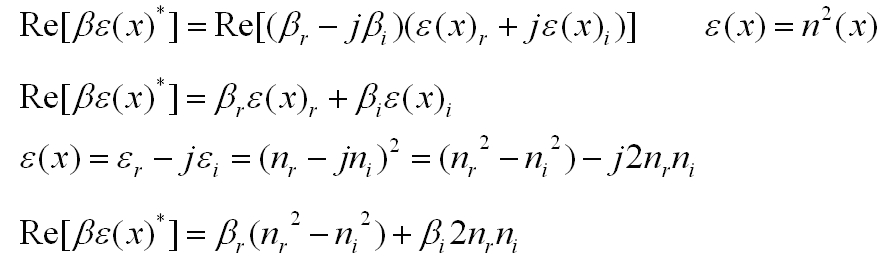where, using the sign convention above, for lossy media, βr >0 and βi >0. For all normal lossy materials (including metals at all frequencies), nr>0 and ni>0. Therefore εi>0 and so βiεi >0. However, εr may be negative and large, when ni > nr as is the case for many metals at optical and infrared frequencies. For example, at λ=1.3um, gold has a complex refractive index n =(0.3 -j8.0) and ε=(-63.9 -j4.8 ). A change in sign of εr between waveguide layers may cause Sz to reverse direction in adjacent waveguide layers, depending on the value of β.

Note that for any medium, including media with loss, a PLANE WAVE will always have the time-averaged power flow Sz in the same direction as the propagation (phase velocity) direction, since any normal lossy medium always has nre >0 (note however that the instantanous power flow for a simple plane wave in a lossy medium may surge strongly between forward and reverse directions during the optical cycle):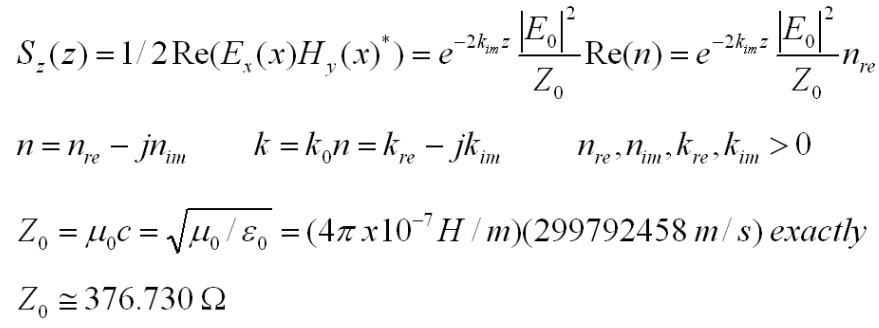Therefore the time-averaged reverse power flow seen in one of the media of the TM surface-wave mode discussed above is a consequence of both a discontinuity in the normal component of the electric vector at the interface along with a sufficiently large negative real part of the dielectric constant in one of the two media. The negative dielectric constant is fundamentally related to the complex phase shift in the electron response in metals due to the driving optical field (Born et. al 1975).

A negative real part of a dielectric constant may not always cause a time-averaged reverse power flow in the conductor of a TM surface-wave mode. The plots below show how the time-averaged relative power flow in the two regions (integrated over the transverse direction x) of a lossless/lossy media TM0 mode configuration changes as the imaginary part of the lossy medium n2.im changes. Note that the real part of the dielectric constant for n2, epsilon2.re becomes negative for n2.im = -0.3 but the x-integrated Sz power flow in that medium Pt2 is still in the phase propagation direction +z. Pt2 only changes direction for n2.im ~ -1.102, at the condition Re(βε*)=0 and remains reversed as n2.im becomes more negative. Note also the peaking in both real and imaginary parts of the mode index n_m with an mode attenuation peak at n2.im ~ -1.3. For very small loss in n2, as n2.im --> 0, both skin depths delta1, delta2 become large as the TM0 surface wave becomes every more unconfined. In the limit of zero loss in n2, the node index becomes exactly the angled-plane-wave configuration at the Brewster angle for an interface between real indices of 1.5 and 0.3 as expected. At the other extreme, as n2.im becomes very large and negative, the mode index approaches that of the lossless medium 1.5, the power is almost completely confined to the lossless region P1t --> 1 with phase fronts in N1 almost normal to the interface with relatively low attenuation and field penetration into the metal n2, P2t --> 0 similar in feature to the well-known behaviour of a good conductor: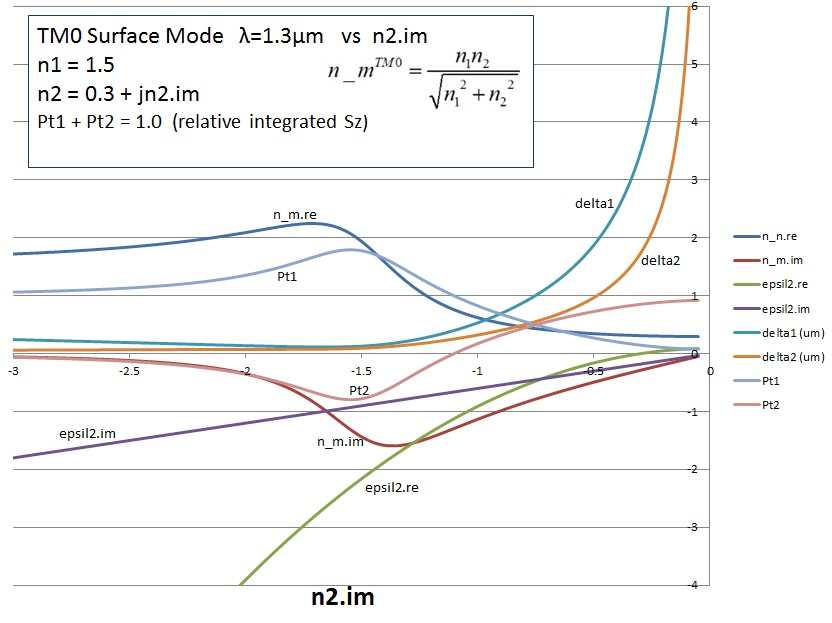The 2D field plots below show the real Hy, Ex, Ez and instantaneous (not time-averaged) Sz Poynting vector components Sz and Sx at the same instant of time for a representative dielectric/metal interface exhibiting strong reverse power flow in the metal (a point at far left side of curve above). The instantaneous Poynting vector view (as compared to the more common time-averaged view) clearly demonstrates the local variation of the energy density on the wavelength scale. The field and Poynting vector patterns move unchanged in shape along the +z propagation direction (the phase velocity direction), except for an overall amplitude attenuation rate of 2βim. The relative phase of the Ex and Hy field components determines the power flow direction. In the metal, for this data point, Ex is close to 180° (163°) out of phase with the Hy field (reversed in sign), while in the dielectric, Ex and Hy are almost exactly in phase. The z component Sz of instantaneous (and also the time averaged) Poynting vector in the metal therefore is in the REVERSE direction (-z) to the phase velocity direction compared to that in the dielectric. The instantaneous Sz profile shows that in fact there is a slight surging into the forward direction (a data point at the transition Re(βε*)=0 would yield a time-averaged Sz=0 everywhere in the metal, but a very strong and balanced instantaneous forwared/reverse surging of Sz with Ex and Hy phase shifted by 90°). Note that the Ez axial field is advanced in phase relative to Hy by about 80°. This leads to an instantaneous forward/reverse surging in power flow across the interface, with a time-averaged net flux into the metal (-x) as can more clearly been seen in the side view of Sx(x,z, t=0) below. The planes of constant phase are tilted slightly in the formward z direction by about 6°: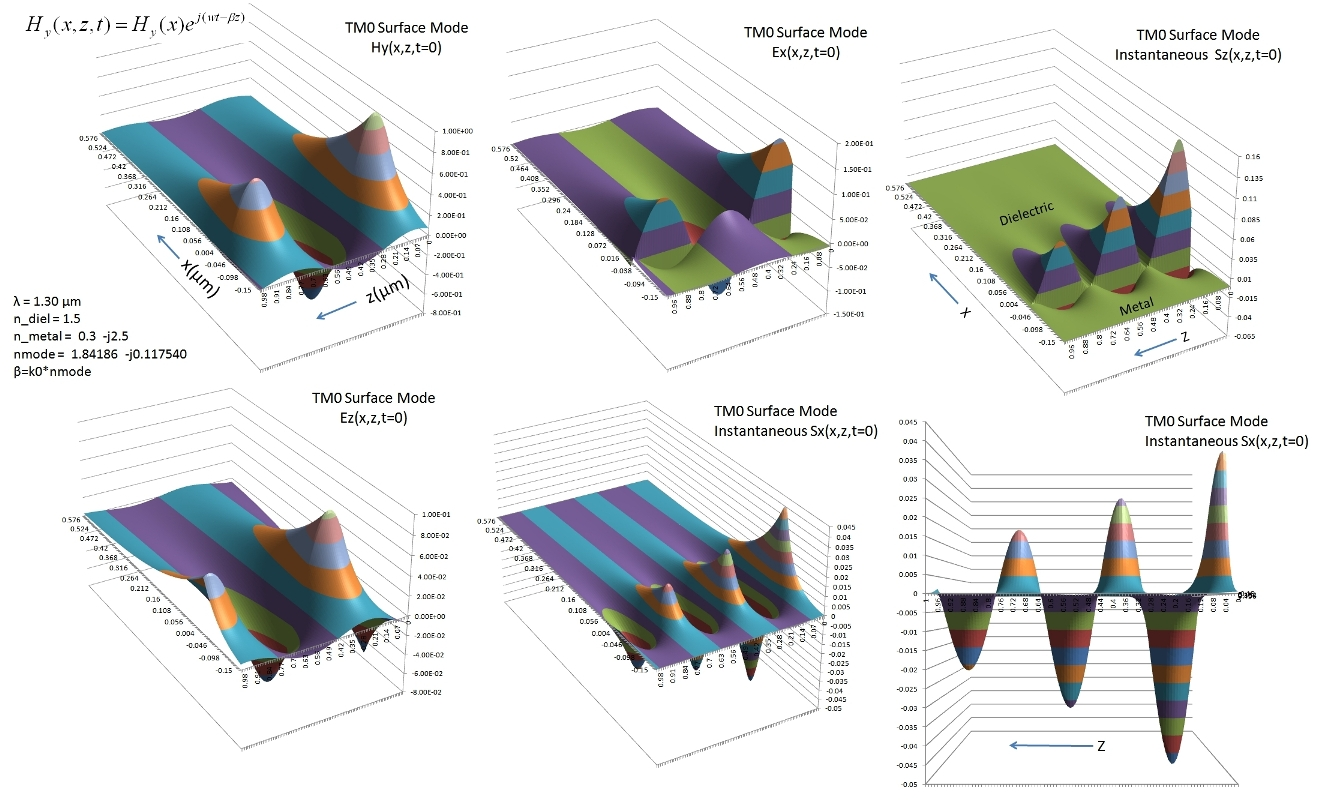The corresponding time-averaged Poynting vector components are shown below at a fixed z and demonstrate the relative values of Sx and Sz. In both cases, the distribution merely attenuates for increasing z. Note that the time-averaged Sx is everywhere negative (flux in the -x direction), showing that the energy flow is toward the metal from the dielectric and into it. The Sz component along the interface shows the flow direction change at the interface as discussed above:It is easy to show using similar analysis to that above that reverse power flow also occurs in the simpler perhaps more intuitive configuration of a P-polarized (transverse magnetic field Hy) simple plane wave reflected/refracted at an interface between a lossless dielectric and a lossy medium with a negative real part of the permittivity. In this case, with an incident and reflected plane wave in the dielectric, with the same orientation as that above for the TM0 surface wave, the component of propagation constant along the interface is real and is β = k0sin(θi) where θi is the real angle of incidence. The z component of Poynting vector along the interface in the real incident medium is in the +z direction. However, again the z component of Poynting vector in the refracted negative permittivity medium is in the -z direction and this is true for ALL real angles of incidence.

Reference:
• Electromagnetic Theory, J. Stratton, 1941, McGraw Hill, pp. 517-524
• Principles of Optics, M. Born, E. Wolf, 5th Edn. 1975, Pergamon Press. pp. 621-626
• An Introduction To Optical Waveguides, M. J. Adams, 1981, J. Wiley & Sons, pp 66-68
• Surface Wave Character On A Slab of Metamaterial with Negative Permittivity and Permeability, S. Mahmoud, A. Viitanen, Progress in Electromagnetics Research, #51, 2005 pp. 127-137